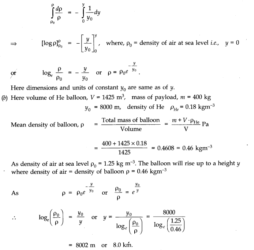# Chapter 10 – Mechanical Properties Of Fluids Questions and Answers: NCERT Solutions for Class 11 Physics

Class 11 Physics NCERT book solutions for Chapter 10 - Mechanical Properties Of Fluids Questions and Answers.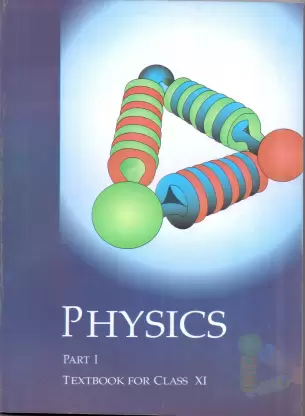## Question 10. 2. Explain why (a) The angle of contact of mercury with glass is obtuse, while that of water with glass is acute. (b) Water on a clean glass surface tends to spread out while mercury on the same surface tends to form drops. (Put differently, water wets glass while mercury does not.) (c) Surface tension of a liquid is independent of the area of the surface. (d) Water with detergent dissolved in it should have small angles of contact. (e) A drop of liquid under no external forces is always spherical in shape.

### Answer:(a) Let a drop of a liquid L be poured on a solid surface S placed in air A. If TSL,and TSAbe the surface tensions corresponding to solid-liquid layer, liquid-air layer and solid-air layer respectively and θ be the angle of contact between the liquid and solid, then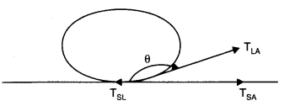## Question 10. 5. A 50 kg girl wearing high heel shoes balances on a single heel. The heel is circular with a diameter 1.0 cm. What is the pressure exerted by the heel on the horizontal floor?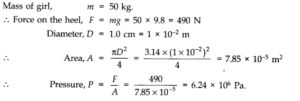## Question 10. 6. Toricelli’s barometer used mercury. Pascal duplicated it using French wine of density 984 kg m-3. Determine the height of the wine column for normal atmospheric pressure.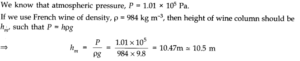## Question 10. 8. A hydraulic automobile lift is designed to lift cars with a maximum mass of 3000 kg. The area of cross-section of the piston carrying the load is 425 cm2. What maximum pressure would the smaller piston have to bear?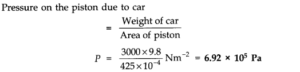## Question 10. 13. Glycerine flows steadily through a horizontal tube of length 1.5 m and radius 1.0 cm. If the amount of glycerine collected per second at one end is 4.0 x 10-3kg s-1, what is the pressure difference between the two ends of the tube? (Density of glycerine = 1.3 x 103kg m-3and viscosity of glycerine = 0.83 Pa s). [You may also like to check if the assumption of laminar flow in the tube is correct],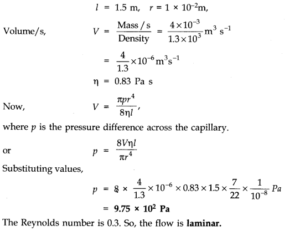## Question 10. 14.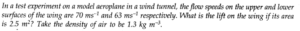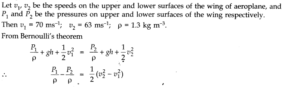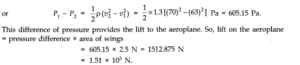## Question 10. 15. Figures (a) and (b) refer to the steady flow of a (non-viscous) liquid. Which of the two figures in incorrect? Why?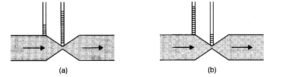## Question 10. 16. The cylindrical tube of a spare pump has a cross-section of 8.0 cm2one end of which has 40 fine holes each of diameter 1.0 mm. If the liquid flow inside the tube is 1.5 m min-1, what is the speed of ejection of the liquid through the holes?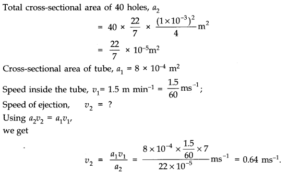## Question 10. 18. Figure (a) below shows a thin film supporting a small weight = 4.5 x 10-2N. What is the weight supported by a film of the same liquid at the same temperature in Fig. (b) and (c) Explain your answer physically.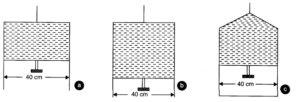## Question 10. 19. What is the pressure inside a drop of mercury of radius 3.0 mm at room temperature? Surface tension of mercury at that temperature (20°C) is 4.65 x 10-1Nm-1. The atmospheric pressure is 1.01 x 105Pa. Also give the excess pressure inside the drop.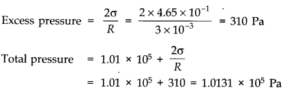## Question 10. 20. What is the excess pressure inside a bubble of soap solution of radius 5.00 mm, given that the surface tension of soap solution at the temperature (20 °C) is 2.50 x 10-2 Nm-1? If an air bubble of the same dimension were formed at depth of 40.0 cm inside a container containing the soap solution (of relative density 1.20), what would be the pressure inside the bubble? (1 atmospheric pressure is 1.01 x 105 Pa).

### Answer:Here surface tension of soap solution at room temperature T = 2.50 x 10-2Nm-1, radius of soap bubble, r = 5.00 mm = 5.00 x 10-3m.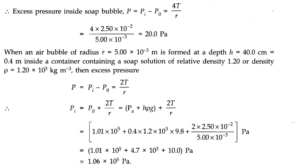## Question 10. 21. A tank with a square base of area 1.0 m2is divided by a vertical partition in the middle. The bottom of the partition has a small-hinged door of area 20 cm2. The tank is filled with water in one compartment, and an acid (of relative density 1.7) in the other, both to a height of 4.0 m. Compute the force necessary to keep the door close.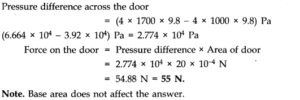## Question 10. 22. A manometer reads the pressure of a gas in an enclosure as shown in Fig. (a) When a pump removes some of the gas, the manometer reads as in Fig. (b). The liquid used in the manometers is mercury and the atmospheric pressure is 76 cm of mercury. (a) Give the absolute and gauge pressure of the gas in the enclosure for cases (a) and (b), in units of cm of mercury. (b) How would the levels change in case (b) if 13.6 cm of water (immiscible with mercury) is poured into the right limb of 1 the manometer? Ignore the small change in the volume of the gas.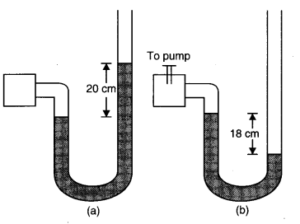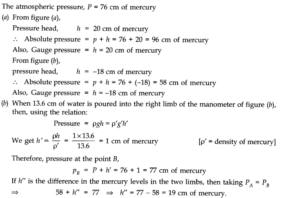## Question 10. 26. (a) What is the largest average velocity of blood flow in an artery of radius 2 x 103m if the flow must remain laminar? (b) What is the corresponding flow rate? Take viscosity of blood to be 2.084 x 10-3Pa-s. Density of blood is 1.06 x 103kg/m3.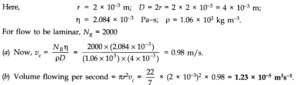## Question 10. 27. A plane is in level flight at constant speed and each of its wings has an area of 25m2. If the speed of the air is 180 km/h over the lower wing and 234 km/h over the upper wing surface, determine the plane’s mass. (Take air density to be 1 kg/m3), g = 9.8 m/s2.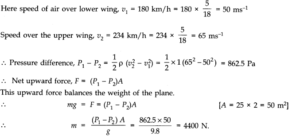## Question 10. 28. In Millikan’s oil drop experiment, what is the terminal speed of an uncharged drop of radius2.0 x 10-5m and density 1.2 x 103kg m-3. Take the viscosity of air at the temperature of the experiment to be 1.8 x 10-5Pa-s. How much is the viscous force on the drop at that speed? Neglect buoyancy of the drop due to air.

### Answer:Here radius of drop, r = 2.0 x 10-5m, density of drop, p = 1.2 x 103kg/m3, viscosity of air TI = 1.8 x 10-5Pa-s. Neglecting upward thrust due to air, we find that terminal speed is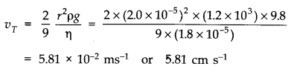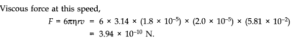## Question 10. 29. Mercury has an angle of contact equal to 140° with soda-lime glass. A narrow tube of radius1.0 mm made of this glass is dipped in a trough containing mercury. By what amount does the mercury dip down in the tube relative to the liquid surface outside? Surface tension of mercury at the temperature of the experiment is 0.465 Nm-2. Density of mercury = 13.6 x 10 kgm-3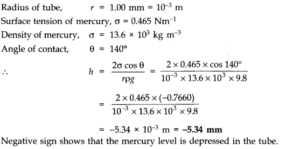## Question 10. 30. Two narrow bores of diameters 3.0 mm and 6.0 mm are joined together to form a U-tube open at both ends. If the U-tube contains water, what is the difference in its levels in the two limbs of the tube? Surface tension of water at the temperature of the experiment is 7.3 x 10-2Nm-2. Take the angle of contact to be zero and density of water to be 1.0 x 103kg m-3(g = 9.8 ms-2).

### Answer:Let rx be the radius of one bore and r2be the radius of second bore of the U-tube. The, if h1 and h2are the heights of water on two sides, then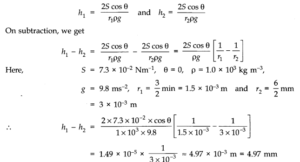## Question 10. 31. (a) It is known that density p of air decreases with height y as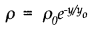## where ρ0= 1.25 kg m-3is the density at sea level, and y0is a constant. This density variation is called the law of atmospheres. Obtain this law assuming that the temperature of atmosphere remains a constant (isothermal conditions). Also, assume that the value of g remains constant. (b) A large He balloon of volume 1425m3is used to lift a payload of 400 kg. Assume that the balloon maintains a constant radius as it rises. How high does it rise?[Take y0= 8000 m and ρHe= 0.18 kg m-3].

### Answer:(a) We know that rate of decrease of density p of air is directly proportional to the height y. It is given as dρ/dy = – ρ/y0 where y is a constant of proportionality and -ve sign signifies that density is decreasing with increase in height. On integration, we get